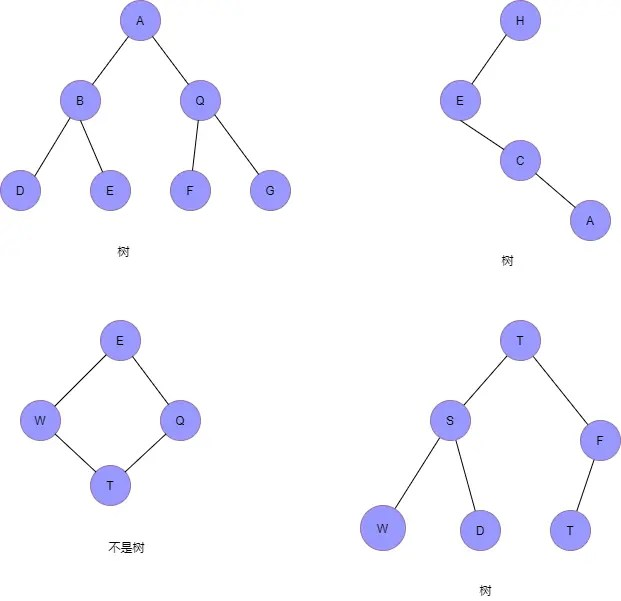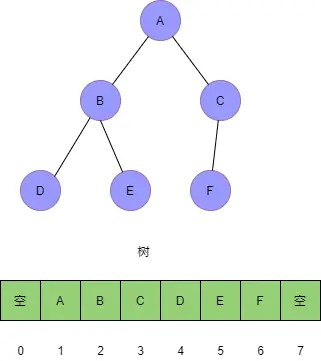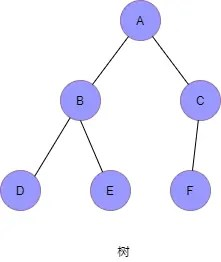# Search Algorithm Series (1) Basics of Binary Tree

Posted Jun 27, 20204 min read

WeChat public account:Xiaochao said

This is the first article in the Find Algorithm series of articles to help you get started with binary trees quickly

## What is a tree?

Let s first look at some pictures:Among them, the first, second, and fourth are all trees, and the third is not. The characteristics of the tree are obvious!

Each of these elements is called a "node"; used to connect the relationship between adjacent nodes, we call it "parent-child relationship". For example, in Figure 1, node A is the parent node of node B, node B is the child node of node A, and at the same time, node B and node Q are child nodes of the same parent node A. Therefore, they become brother nodes. We call the node without the parent node the root node, which is the A node in Figure 1. We call the nodes without child nodes leaf nodes, such as the D, E, F, and G nodes in Figure 1. These concepts are obvious, but they are the most basic things.

## Binary tree

A binary tree is naturally a tree with at most two branches per node, that is, a tree with two child nodes. The two branches are called left subtree and right subtree. Taking the above picture as an example, Figure 1, Figure 2 and Figure 4 are all binary trees, because each of them contains at most two child nodes. Among them, Figure 1 is also called full binary tree, and Figure 4 is also called complete binary tree. The reason why the complete binary tree appears is actually based on the physical storage method of the binary tree.

#### Binary tree storage method

• Chain storage method based on linked list
• Array-based sequential storage method

Chain storage method:

We create a Node object for each node:

``````class Node{
int data;
Node left,right;
}``````

Each node is a Node object, which contains the data we need to store, the reference to the left child node, and the reference to the right child node, just like a linked list, the entire tree is stringed together. If the node has no left child nodes, then `Node.left==null` or `Node.right==null`.

Sequential storage method:

We store the root node at the position with subscript `i=1`, then the left child node is stored at `2*i=2`, the right child node is stored at the subscript`2*i+1=2` . And so on, complete the storage of the tree. With the help of subscript operations, we can easily jump from the parent node to the left child node and the right child node or find its parent node from any child node. If the position of X is i, then the subscripts of its two child nodes are `2i` and `2i+1`, respectively, and the position of its parent node is `i/2`(here the result is rounded down).

As shown in the following figure:It can be found that only the complete binary tree storage has the highest efficiency and the most memory saving#### Traversal of Binary Tree

There are three main traversal operations for binary trees

• Preorder traversal
• In-order traversal
• Post-order traversal

Preorder traversal means that for any node in the tree, print this node first, then print its left subtree, and finally print its right subtree.

Mid-order traversal means that for any node in the tree, first print its left subtree, then print itself, and finally print its right subtree.

Post-order traversal means that for any node in the tree, first print its left subtree, then print its right subtree, and finally print the node itself.

Note that this has a recursive taste

Take the picture just now as an example:• Preorder traversal:A->B->D->E->C->F
• Mid-order traversal:D->B->E->A->F->C
• Post-order traversal:D->E->B->F->C->A

Specific code implementation(just write recursion):

``````public void preOrder(Node root){
if(root==null) return;
System.out.println(root.data);//Print the value of the root node
preOrder(root.left);
preOrder(root.right);
}

public void inOrder(Node root){
if(root==null) return;
inOrder(root.left);
Systrm.out.println(root.data);
inOrder(root.right);
}

public void inOrder(Node root){
if(root==null) return;
inOrder(root.left)
inOrder(root.right);
Systrm.out.println(root.data);
}``````

The time complexity of binary tree traversal is O(n), this is because each node will be accessed at most twice,(the function is pushed into and out of the stack when recursive), so the number of visits of the traversal operation is proportional to the number of nodes n , That is to say, the time complexity of binary tree traversal is O(n).

## Outlook

After the above introduction, we already have a basic understanding of the binary tree. So, what is the significance of the existence of the binary tree? What efficient algorithms can we design based on this data structure? Next time we will introduce Binary Search Tree(Binary Search Tree), we will define a data structure and maintain its properties.

Off-topic:For beginners in algorithms, I recommend a very nice book "Fourth Edition of Algorithms", where the various maps are very detailed. If you need an electronic version of the file, reply Algorithm 4 in the background to get the download link. Reply in the background Algorithm 01 Send you a mind map of algorithm and data structure. Finally, I hope we can make progress together and grow together!